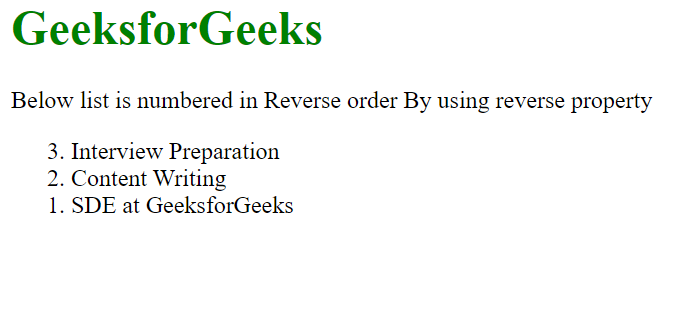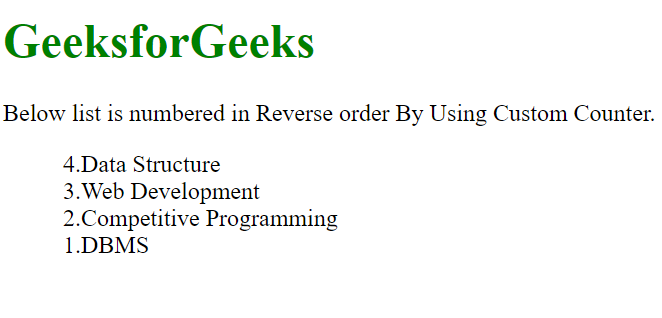Open in App
Not now

# How to Reverse Custom Counters using CSS ?

• Last Updated : 25 Aug, 2021

Counters in CSS are basically variables which can be used for numbering and values of CSS counters may be incremented by CSS rules. Follow the article, you will get to know 2 methods to reverse custom counter using CSS.

We will be going to use the following two approaches to reverse counters:

• In approach 1 we will use how to reverse simple counter using reverse property in HTML.
• In approach 2 we will finally solve our problem statement on how to reverse Custom Counters using CSS.

Approach 1: Reverse counter using reversed attribute in HTML.

Firstly, we will assume that we know the total no.of items in the list because we will instruct the counter to start numbering from total no.of items and keeps on decreasing by one till 0(zero) and through this we can achieve the numbering in reverse order.

Note: The HTML <ol> reversed Attribute is a Boolean Attribute and used to ordered the list in Descending Order(9, 8, 7, 6 …..) instead of ascending order(1, 2, 3 ….).

Syntax:

```<ol reversed>
<li> Content... </li>
<li> Content... </li>
...
<li> Content... </li>
</ol>```

Below is the implementation of the above approach.

Example:

## HTML

 ```<``html``>`` ` `<``body``>``    ``<``h1` `style``=``"color: green;"``>``        ``GeeksforGeeks``    ```` ` `    ` `<``p``>``        ``Below list is numbered in Reverse``        ``order By using reverse property``    ```  ` ` `    ``<``ol` `reversed>``        ``<``li``>Interview Preparation``        ``<``li``>Content Writing``        ``<``li``>SDE at GeeksforGeeks``    `````` ` ``

Output:Above example is not a custom counter. It is a built-in method to reverse the counter. Follow the below approach to customize the order of the counter.

Approach 2: Using custom counter to reverse a counter. Below is the step-by-step implementation on how to use custom counter.

Step 1: At first need to disable the default counters so that no marker will be shown and this can be done by setting the CSS property list-style-type to none.

Step 2: We need to create a counter in CSS to track the number of items in the list using counter-reset which creates or resets a counter and set the counter-reset  = ( total number of element in the list + 1). Adding one more element is due to counter() rule.

`counter-reset: myCounter (total number of element in the list + 1);`

Step 3: Use the counter() function in a content which is used to display the content in a particular order.

`content: counter(myCounter);`

Step 4: At last, to increment the CSS counter. You need to add counter-increment Property which is used to increment/decrement value of a counter and set the counter-increment = -1 , which use to decrease the counter by 1.Actually counter-increment property increases the integer by 1, but here we will alter this property to decrease the integer by 1.

`counter-increment: myCounter -1;`

Example 2:

## HTML

 ```<``html``>`` ` `<``head``>``    ``<``style``>``        ``h1 {``            ``color: green;``        ``}`` ` `        ``/* STEP-1 and STEP-2 */``        ``ol {`` ` `            ``/* Disable the default counters  */``            ``list-style-type: none;`` ` `            ``/* Creates a counter  */``            ``counter-reset: myCounter 5;``        ``}`` ` `        ``/* STEP-3 and STEP-4 */``        ``ol li::before {`` ` `            ``/* To display the content in``               ``a particular order. */``            ``content: counter(myCounter) ".";`` ` `            ``/* Decrease the counter by 1.*/``            ``counter-increment: myCounter -1;``        ``}``    `````` ` `<``body``>``    ``<``h1``>GeeksforGeeks`` ` `    ` `<``p``>``        ``Below list is numbered in Reverse``        ``order By Using Custom Counter.``    ```  ` ` `    ``<``ol` `class``=``"counter"``>``        ``<``li``>Data Structure``        ``<``li``>Web Development``        ``<``li``>Competitive Programming``        ``<``li``>DBMS``    `````` ` ``

Output:My Personal Notes arrow_drop_up